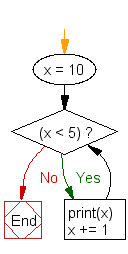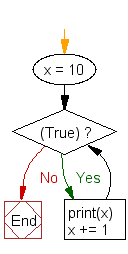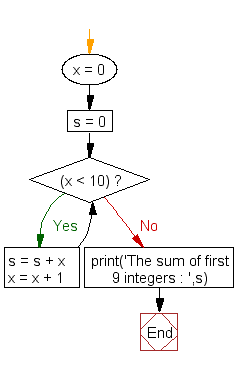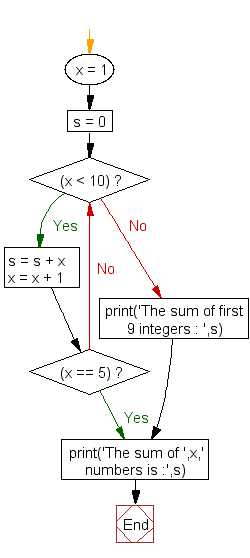# Python while loop

## While loop

Loops are used to repeatedly execute a block of program statements. The basic loop structure in Python is while loop. Here is the syntax.

Syntax:

while (expression) :
statement_1
statement_2
....

The while loop runs as long as the expression (condition) evaluates to True and execute the program block. The condition is checked every time at the beginning of the loop and the first time when the expression evaluates to False, the loop stops without executing any remaining statement(s). The following example prints the digits 0 to 4 as we set the condition x < 5.

``````x = 0;
while (x < 5):
print(x)
x += 1
``````

Output:

```0
1
2
3
4```

One thing we should remember that a while loop tests its condition before the body of the loop (block of program statements) is executed. If the initial test returns false, the body is not executed at all. For example the following code never prints out anything since before executing the condition evaluates to false.

``````x = 10;
while (x < 5):
print(x)
x += 1
``````

Flowchart:The following while loop is an infinite loop, using True as the condition:

``````x = 10;
while (True):
print(x)
x += 1
``````

Flowchart:## Python: while and else statement

There is a structural similarity between while and else statement. Both have a block of statement(s) which is only executed when the condition is true. The difference is that block belongs to if statement executes once whereas block belongs to while statement executes repeatedly.

You can attach an optional else clause with while statement, in this case, syntax will be -

while (expression) :
statement_1
statement_2
......
else :
statement_3
statement_4
......

The while loop repeatedly tests the expression (condition) and, if it is true, executes the first block of program statements. The else clause is only executed when the condition is false it may be the first time it is tested and will not execute if the loop breaks, or if an exception is raised. If a break statement executes in first program block and terminates the loop then the else clause does not execute. In the following example, while loop calculates the sum of the integers from 0 to 9 and after completing the loop, else statement executes.

``````
x = 0;
s = 0
while (x < 10):
s = s + x
x = x + 1
else :
print('The sum of first 9 integers : ',s)
``````

Output:

`The sum of first 9 integers:  45`

Flowchart:## Example: while loop with if-else and break statement

``````x = 1;
s = 0
while (x < 10):
s = s + x
x = x + 1
if (x == 5):
break
else :
print('The sum of first 9 integers : ',s)
print('The sum of ',x,' numbers is :',s)
``````

Output:

`The sum of  5  numbers is : 10`

In the above example the loop is terminated when x becomes 5. Here we use break statement to terminate the while loop without completing it, therefore program control goes to outside the while - else structure and execute the next print statement.

Flowchart:Previous: Python For Loop
Next: Python break, continue

Test your Python skills with w3resource's quiz

﻿

## Python: Tips of the Day

Clamps num within the inclusive range specified by the boundary values x and y:

Example:

```def tips_clamp_num(num,x,y):
return max(min(num, max(x, y)), min(x, y))
print(tips_clamp_num(2, 4, 6))
print(tips_clamp_num(1, -1, -6))
```

Output:

```4
-1
```